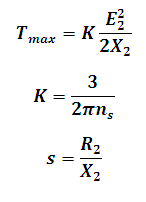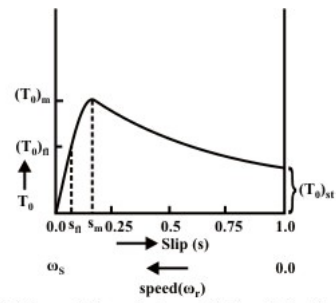# Maximum Torque in Induction Motor Calculator

This Calctown Calculator calculates the maximum torque that the motor is capable of producing and the slip for maximum torque.

ohm
ohm

#### Result

Nmwhere

E2 = rotor emf

Tmax = max torque

X2 = rotor reactance

R2 = rotor resistance

s = slip

ns = synchronous speed in rps